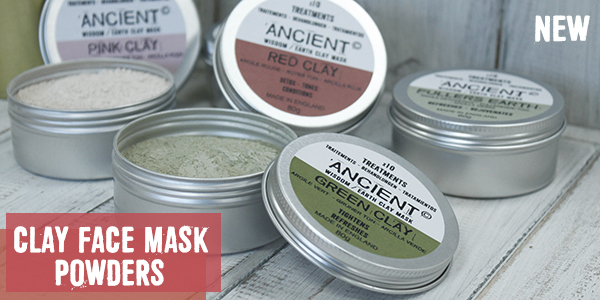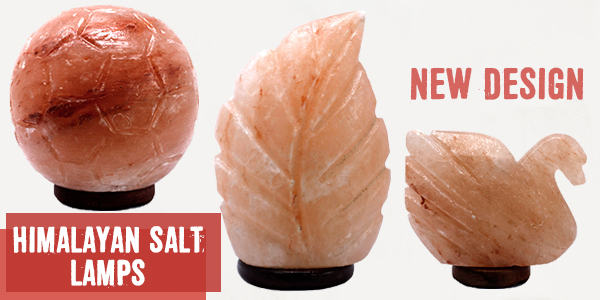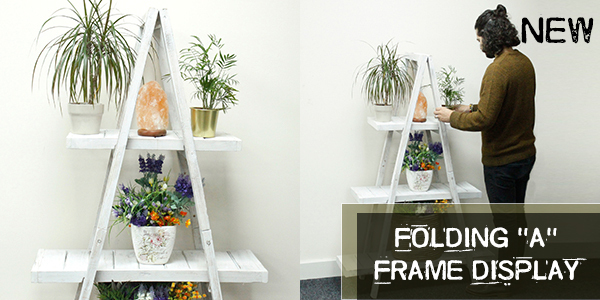Like   Tweet   Pin   +1   in
 table div table+table+table div table td,table.module-2{width:100%;padding:0}table div table+table+table div table{width:100%;float:none;margin-left:auto;margin-right:auto;padding:0}table div table+table+table div table a{border:0 none;text-decoration:none}table div table+table+table div table img{width:100%!important;border:0 none;text-decoration:none}/* styles */
 /* styles */ Wholesale Solid Shampoo base is made from high-quality vegetable oils it creates a wonderfully dense creamy lather, which is naturally conditioning to the hair. They are also environmentally friendly as you are not wasting bottles and packaging and paying for water to be shipped around the country.Wholesale Clay Face Mask Powders
 /* styles */ For centuries, clay has been used for medicinal and cosmetic purposes in many cultures. Nowadays, clay face masks are used as one of the most effective treatments for skin problems. Here we have the most popular Clay Face Mask powders, ideal to sell along side Floral Waters, see below. Perfect for pamper sets, a team with brushes and scrubs for a perfect gift.
 table div table+table+table+table+table+table+table div table td,table.module-6{width:100%;padding:0}table div table+table+table+table+table+table+table div table{width:100%;float:none;margin-left:auto;margin-right:auto;padding:0}table div table+table+table+table+table+table+table div table a{border:0 none;text-decoration:none}table div table+table+table+table+table+table+table div table img{width:100%!important;border:0 none;text-decoration:none}/* styles */
 /* styles */ This Nepali Pure Herbs Incense Ropes are made up of herbs ie. found in the high Himalayas. It is used in Stupas, Temples and drawing rooms for aromatic ambience.NEW Designs Lamps & Candle Holders
 /* styles */ Each Wholesale Salt Lamp comes complete with light fitting, bulb & plug. Rather than sell them by size we sell them by approximate weight. Items are marked with sizes to give a rough idea of size only, each lamp is completely unique.
 table div table+table+table+table+table+table+table+table+table+table+table div table td,table.module-10{width:100%;padding:0}table div table+table+table+table+table+table+table+table+table+table+table div table{width:100%;float:none;margin-left:auto;margin-right:auto;padding:0}table div table+table+table+table+table+table+table+table+table+table+table div table a{border:0 none;text-decoration:none}table div table+table+table+table+table+table+table+table+table+table+table div table img{width:100%!important;border:0 none;text-decoration:none}/* styles */
 /* styles */ Wooden Soap Dishes back in stock, nice modern designs, very well made and all packed in individual polypropylene bags and also great value wholesale wooden soap dishes.
 table div table+table+table+table+table+table+table+table+table+table+table+table+table div table td,table.module-12{width:100%;padding:0}table div table+table+table+table+table+table+table+table+table+table+table+table+table div table{width:100%;float:none;margin-left:auto;margin-right:auto;padding:0}table div table+table+table+table+table+table+table+table+table+table+table+table+table div table a{border:0 none;text-decoration:none}table div table+table+table+table+table+table+table+table+table+table+table+table+table div table img{width:100%!important;border:0 none;text-decoration:none}/* styles */
 /* styles */ All year round skin needs exfoliating but especially in the winter as it is when skin is the driest and with our brush you can get that clean luxurious feeling and keep your skin feeling soft to the touch.Keep your skin feeling radiant even in the cold weather with our wholesale Long Handle Body Brush
 table div table+table+table+table+table+table+table+table+table+table+table+table+table+table+table div table td,table.module-14{width:100%;padding:0}table div table+table+table+table+table+table+table+table+table+table+table+table+table+table+table div table{width:100%;float:none;margin-left:auto;margin-right:auto;padding:0}table div table+table+table+table+table+table+table+table+table+table+table+table+table+table+table div table a{border:0 none;text-decoration:none}table div table+table+table+table+table+table+table+table+table+table+table+table+table+table+table div table img{width:100%!important;border:0 none;text-decoration:none}/* styles */
 /* styles */ These are easy to use, light and compact-sized Wholesale wooden massagers means your customers can carry them around them and give themselves a massage whenever they feel the urge and can do so even at the office.Folding "A" Frame Display - Whitewash
 /* styles */ This modern on-trend A-frame display in whitewash colours is perfect for creating an eye-catch in your store and displaying your lovely products. This is also great for using as a room divider, add some pot plants and it will look amazing. With its contemporary modern design, this frame display can be placed in any room of your customer’s house.
 table div table+table+table+table+table+table+table+table+table+table+table+table+table+table+table+table+table+table+table div table td,table.module-18{width:100%;padding:0}table div table+table+table+table+table+table+table+table+table+table+table+table+table+table+table+table+table+table+table div table{width:100%;float:none;margin-left:auto;margin-right:auto;padding:0}table div table+table+table+table+table+table+table+table+table+table+table+table+table+table+table+table+table+table+table div table a{border:0 none;text-decoration:none}table div table+table+table+table+table+table+table+table+table+table+table+table+table+table+table+table+table+table+table div table img{width:100%!important;border:0 none;text-decoration:none}/* styles */
 /* styles */ Wholesale Oil Burners is growing all the time. One of the best wholesale oil burners ranges available and all at keen prices. We import from India and China and stock all the latest design trends. It’s worth noting that a grand selection of oil burners is a great way to build sales of related items.
 table div table+table+table+table+table+table+table+table+table+table+table+table+table+table+table+table+table+table+table+table+table div table td,table.module-20{width:100%;padding:0}table div table+table+table+table+table+table+table+table+table+table+table+table+table+table+table+table+table+table+table+table+table div table{width:100%;float:none;margin-left:auto;margin-right:auto;padding:0}table div table+table+table+table+table+table+table+table+table+table+table+table+table+table+table+table+table+table+table+table+table div table a{border:0 none;text-decoration:none}table div table+table+table+table+table+table+table+table+table+table+table+table+table+table+table+table+table+table+table+table+table div table img{width:100%!important;border:0 none;text-decoration:none}/* styles */
 /* styles */ They are 21cm non drip and fit most candle holders. Candles are of 'church candle' quality, over-dipped with colour. Made in Germany to an exacting standard, they burn for approximately 8 hours with a tall clean flame.
 table div table+table+table+table+table+table+table+table+table+table+table+table+table+table+table+table+table+table+table+table+table+table+table div table td,table.module-22{width:100%;padding:0}table div table+table+table+table+table+table+table+table+table+table+table+table+table+table+table+table+table+table+table+table+table+table+table div table{width:100%;float:none;margin-left:auto;margin-right:auto;padding:0}table div table+table+table+table+table+table+table+table+table+table+table+table+table+table+table+table+table+table+table+table+table+table+table div table a{border:0 none;text-decoration:none}table div table+table+table+table+table+table+table+table+table+table+table+table+table+table+table+table+table+table+table+table+table+table+table div table img{width:100%!important;border:0 none;text-decoration:none}/* styles */
 /* styles */ These Natural deodorants are not processed in any way except they are carved from natural chunks of Himalayas Crystals. The deodorant stone leaves a layer of protection that helps prevent odour causing bacteria from forming.
 table div table+table+table+table+table+table+table+table+table+table+table+table+table+table+table+table+table+table+table+table+table+table+table+table+table div table td,table.module-24{width:100%;padding:0}table div table+table+table+table+table+table+table+table+table+table+table+table+table+table+table+table+table+table+table+table+table+table+table+table+table div table{width:100%;float:none;margin-left:auto;margin-right:auto;padding:0}table div table+table+table+table+table+table+table+table+table+table+table+table+table+table+table+table+table+table+table+table+table+table+table+table+table div table a{border:0 none;text-decoration:none}table div table+table+table+table+table+table+table+table+table+table+table+table+table+table+table+table+table+table+table+table+table+table+table+table+table div table img{width:100%!important;border:0 none;text-decoration:none}/* styles */
 /* styles */ These brazier sets are sold in 3 different shapes with Salt rocks or without them to suit best your customer's needs. Each Wholesale Salt Rock Brazier Set comes complete with light fitting, bulb, plug and 3kg salt chunks or without the salt chunks.
 table div table+table+table+table+table+table+table+table+table+table+table+table+table+table+table+table+table+table+table+table+table+table+table+table+table+table+table div table td,table.module-26{width:100%;padding:0}table div table+table+table+table+table+table+table+table+table+table+table+table+table+table+table+table+table+table+table+table+table+table+table+table+table+table+table div table{width:100%;float:none;margin-left:auto;margin-right:auto;padding:0}table div table+table+table+table+table+table+table+table+table+table+table+table+table+table+table+table+table+table+table+table+table+table+table+table+table+table+table div table a{border:0 none;text-decoration:none}table div table+table+table+table+table+table+table+table+table+table+table+table+table+table+table+table+table+table+table+table+table+table+table+table+table+table+table div table img{width:100%!important;border:0 none;text-decoration:none}/* styles */
 /* styles */ High-quality wholesale wrapping tissue paper great for a whole host of applications from general wrapping and interleaving to void filling and craft use.
 table div table+table+table+table+table+table+table+table+table+table+table+table+table+table+table+table+table+table+table+table+table+table+table+table+table+table+table+table+table div table td,table.module-28{width:100%;padding:0}table div table+table+table+table+table+table+table+table+table+table+table+table+table+table+table+table+table+table+table+table+table+table+table+table+table+table+table+table+table div table{width:100%;float:none;margin-left:auto;margin-right:auto;padding:0}table div table+table+table+table+table+table+table+table+table+table+table+table+table+table+table+table+table+table+table+table+table+table+table+table+table+table+table+table+table div table a{border:0 none;text-decoration:none}table div table+table+table+table+table+table+table+table+table+table+table+table+table+table+table+table+table+table+table+table+table+table+table+table+table+table+table+table+table div table img{width:100%!important;border:0 none;text-decoration:none}/* styles */
 /* styles */ Our warehouse & offices are closed from Friday midday 21st December 2018 - Monday 1st January 2019 inclusive. Naturally, you can place orders online at any time (24/7/365) including over the Christmas period.